# Greybox

#### 2020-02-08

There are three well-known notions of “boxes” in modelling: 1. White box - the model that is completely transparent and does not have any randomness. One can see how the inputs are transformed into the specific outputs. 2. Black box - the model which does not have an apparent structure. One can only observe inputs and outputs but does not know what happens inside. 3. Grey box - the model that is in between the first two. We observe inputs and outputs plus have some information about the structure of the model, but there is still a part of unknown.

The white boxes are usually used in optimisations (e.g. linear programming), while black boxes are popular in machine learning. As for the grey box models, they are more often used in analysis and forecasting. So the package greybox contains models that are used for these purposes.

At the moment the package contains advanced linear model function and several basic functions that implement model selection and combinations using information criteria (IC). You won’t find statistical tests in this package - there’s plenty of them in the other packages. Here we try using the modern techniques and methods that do not rely on hypothesis testing. This is the main philosophical point of greybox.

## Main functions

The package includes the following functions for models construction:

1. alm() - Advanced Linear Model. This is something similar to GLM, but with a focus on forecasting and the information criteria usage for time series. It also supports mixture distribution models for the intermittent data.
2. stepwise() - select the linear model with the lowest IC from all the possible in the provided data. Uses partial correlations. Works fast;
3. lmCombine() - combine the linear models into one using IC weights;
4. lmDynamic() - produce model with dynamic weights and time varying parameters based on IC weight.

See discussion of some of these functions in this vignette below.

## Models evaluation functions

1. ro() - produce forecasts with a specified function using rolling origin.
2. rmc() - regression for multiple comparison of methods. This is a parametric counterpart of nemenyi(), which is more informative than nemenyi() and should work better on large samples. This can be efficiently used together with RelMAE / RelMSE measures.
3. measures() - function, returning a bunch of error measures for the provided forecast and the holdout sample.

## Marketing analytics tools

1. tableplot() - creates a plot for two categorical variables based on table function with frequencies inside.
2. cramer() - Cramer’s V value.
3. mcor() - the multiple correlation coefficient.
4. association() - the matrix of measures of association.
5. spread() - function that plots scatter / boxplot / tableplot diagrams between variables depending on the their types.
6. determination() - coefficients of determination for the set of explanatory variables.

All these functions are discussed in a separate vignette on marketing analytics tools.

## Methods

The following methods can be applied to the models, produced by alm(), stepwise(), lmCombine() and lmDynamic(): 1. logLik() - extracts log-likelihood. 2. AIC(), AICc(), BIC(), BICc() - calculates the respective information criteria. 3. pointLik() - extracts the point likelihood. 4. pAIC(), pAICc(), pBIC(), pBICc() - calculates the respective point information criteria, based on pointLik. 5. actuals() - extracts the actual values of the response variable. 6. coef(), coefficients() - extract the parameters of the model. 7. confint() - extracts the confidence intervals for the parameters. 8. vcov() - extracts the variance-covariance matrix of the parameters. 9. sigma() - extracts the standard deviation of the residuals. 10. nobs() - the number of the in-sample observations of the model. 11. nparam() - the number of all the estimated parameters in the model. 12. summary() - produces the summary of the model. 13. predict() - produces the predictions based on the model and the provided newdata. If the newdata is not provided, then it uses the already available data in the model. Can also produce confidence and prediction intervals. 14. forecast() - acts similarly to predict() with few differences. It has a parameter h - forecast horizon - which is NULL by default and is set to be equal to the number of rows in newdata. However, if the newdata is not provided, then it will produce forecasts of the explanatory variables to the horizon h and use them as newdata. Finally, if h and newdata are provided, then the number of rows to use will be regulated by h. 15. plot() - produces several plots for the analysis of the residuals. This includes: Fitted over time, Standardised residuals vs Fitted, Absolute residuals vs Fitted, Q-Q plot with the specified distribution, Squared residuals vs Fitted, ACF of the residuals and PACF of the residuals, which is regulated by which parameter. See documentation for more info: ?plot.greybox.

## Exogenous variables transformation tools

1. xregExpander() - expand the provided data by including leads and lags of the variables.
2. xregTransformer() - produce non-linear transformations of the provided data (logs, inverse etc).
3. xregMultiplier() - produce cross-products of the variables in the provided matrix. Could be useful when exploring interaction effects of dummy variables.

See details on (1) below.

## Distribution functions

1. qlaplace(), dlaplace(), rlaplace(), plaplace() - functions for Laplace distribution.
2. qalaplace(), dalaplace(), ralaplace(), palaplace() - functions for Asymmetric Laplace distribution.
3. qs(), ds(), rs(), ps() - functions for S distribution.
4. qfonrm(), dfnorm(), rfnorm(), pfnorm() - functions for folded normal distribution.
5. qtplnorm(), dtplnorm(), rtplnorm(), ptplnorm() - functions for three parameter log normal distribution.
6. qbcnorm(), dbcnorm(), rbcnorm(), pbcnorm() - functions for the Box-Cox normal distribution.

1. graphmaker() - produces linear plots for the variable, its forecasts and fitted values.

The first two construct a model of a class lm, that could be used for the purposes of analysis or forecasting. The last one expands the exogenous variables to the matrix with lags and leads. Let’s see how all of them work. Let’s start from the end.

## xregExpander

The function xregExpander() is useful in cases when the exogenous variable may influence the response variable either via some lags or leads. As an example, consider BJsales.lead series from the datasets package. Let’s assume that the BJsales variable is driven by the today’s value of the indicator, the value five and 10 days ago. This means that we need to produce lags of BJsales.lead. This can be done using xregExpander():

BJxreg <- xregExpander(BJsales.lead,lags=c(-5,-10))

The BJxreg is a matrix, which contains the original data, the data with the lag 5 and the data with the lag 10. However, if we just move the original data several observations ahead or backwards, we will have missing values in the beginning / end of series, so xregExpander() fills in those values with the forecasts using es() and iss() functions from smooth package (depending on the type of variable we are dealing with). This also means that in cases of binary variables you may have weird averaged values as forecasts (e.g. 0.7812), so beware and look at the produced matrix. Maybe in your case it makes sense to just substitute these weird numbers with zeroes…

You may also need leads instead of lags. This is regulated with the same lags parameter but with positive values:

BJxreg <- xregExpander(BJsales.lead,lags=c(7,-5,-10))

Once again, the values are shifted, and now the first 7 values are backcasted. In order to simplify things we can produce all the values from 10 lags till 10 leads, which returns the matrix with 21 variables:

BJxreg <- xregExpander(BJsales.lead,lags=c(-10:10))

## stepwise

The function stepwise() does the selection based on an information criterion (specified by user) and partial correlations. In order to run this function the response variable needs to be in the first column of the provided matrix. The idea of the function is simple, it works iteratively the following way:

1. The basic model of the first variable and the constant is constructed (this corresponds to simple mean). An information criterion is calculated;
2. The correlations of the residuals of the model with all the original exogenous variables are calculated;
3. The regression model of the response variable and all the variables in the previous model plus the new most correlated variable from (2) is constructed using lm() function;
4. An information criterion is calculated and is compared with the one from the previous model. If it is greater or equal to the previous one, then we stop and use the previous model. Otherwise we go to step 2.

This way we do not do a blind search, going forward or backwards, but we follow some sort of “trace” of a good model: if the residuals contain a significant part of variance that can be explained by one of the exogenous variables, then that variable is included in the model. Following partial correlations makes sure that we include only meaningful (from technical point of view) variables in the model. In general the function guarantees that you will have the model with the lowest information criterion. However this does not guarantee that you will end up with a meaningful model or with a model that produces the most accurate forecasts. So analyse what you get as a result.

Let’s see how the function works with the Box-Jenkins data. First we expand the data and form the matrix with all the variables:

BJxreg <- as.data.frame(xregExpander(BJsales.lead,lags=c(-10:10)))
BJxreg <- cbind(as.matrix(BJsales),BJxreg)
colnames(BJxreg) <- "y"
ourModel <- stepwise(BJxreg)

This way we have a nice data frame with nice names, not something weird with strange long names. It is important to note that the response variable should be in the first column of the resulting matrix. After that we use stepwise function:

ourModel <- stepwise(BJxreg)

And here’s what it returns (the object of class lm):

ourModel
#> Call:
#> alm(formula = y ~ xLag4 + xLag9 + xLag3 + xLag6 + xLag10 + xLag5 +
#>     xLead10 + xLag7 + xLag8, data = data, distribution = "dnorm")
#>
#> Coefficients:
#> (Intercept)       xLag4       xLag9       xLag3       xLag6      xLag10
#>  18.6447953   3.3634480   1.3417443   4.6249234   1.6857044   1.5309053
#>   2.3120137   0.3946406   1.3990944   1.3745206

The values in the function are listed in the order of most correlated with the response variable to the least correlated ones. The function works very fast because it does not need to go through all the variables and their combinations in the dataset.

All the basic methods can be used together with the final model (e.g. predict(), forecast(), summary() etc).

## lmCombine

lmCombine() function creates a pool of linear models using lm(), writes down the parameters, standard errors and information criteria and then combines the models using IC weights. The resulting model is of the class “lm.combined”. The speed of the function deteriorates exponentially with the increase of the number of variables $$k$$ in the dataset, because the number of combined models is equal to $$2^k$$. The advanced mechanism that uses stepwise() and removes a large chunk of redundant models is also implemented in the function and can be switched using bruteforce parameter.

Here’s an example of the reduced data with combined model and the parameter bruteforce=TRUE:

ourModel <- lmCombine(BJxreg[,-c(3:7,18:22)],bruteforce=TRUE)
summary(ourModel)
#> The AICc combined model
#> Response variable: y
#> Distribution used in the estimation: Normal
#> Coefficients:
#>             Estimate Std. Error Importance Lower 2.5% Upper 97.5%
#> (Intercept)  21.4896     0.2342     1.0000    21.0266     21.9525
#> x            -0.0499     0.0291     0.2583    -0.1074      0.0077
#> xLag5         6.3926     0.0847     1.0000     6.2252      6.5601
#> xLag4         5.8349     0.0907     1.0000     5.6555      6.0143
#> xLag3         5.6701     0.0909     1.0000     5.4903      5.8499
#> xLag2         0.1188     0.0374     0.2819     0.0449      0.1927
#> xLag1        -0.0893     0.0349     0.2710    -0.1583     -0.0203
#> xLead1       -0.0957     0.0331     0.2779    -0.1611     -0.0304
#> xLead2       -0.0358     0.0258     0.2576    -0.0868      0.0152
#> xLead3       -0.1323     0.0363     0.3013    -0.2040     -0.0606
#> xLead4        0.0065     0.0229     0.2565    -0.0388      0.0517
#> xLead5        0.1248     0.0314     0.3065     0.0626      0.1869
#>
#> Error standard deviation: 2.216
#> Sample size: 150
#> Number of estimated parameters: 7.211
#> Number of degrees of freedom: 142.789
#> Approximate combined information criteria:
#>      AIC     AICc      BIC     BICc
#> 671.8449 672.6800 693.5546 695.6469

summary() function provides the table with the parameters, their standard errors, their relative importance and the 95% confidence intervals. Relative importance indicates in how many cases the variable was included in the model with high weight. So, in the example above variables xLag5, xLag4, xLag3 were included in the models with the highest weights, while all the others were in the models with lower ones. This may indicate that only these variables are needed for the purposes of analysis and forecasting.

The more realistic situation is when the number of variables is high. In the following example we use the data with 21 variables. So if we use brute force and estimate every model in the dataset, we will end up with $$2^{21}$$ = 2^21 combinations of models, which is not possible to estimate in the adequate time. That is why we use bruteforce=FALSE:

ourModel <- lmCombine(BJxreg,bruteforce=FALSE)
summary(ourModel)
#> The AICc combined model
#> Response variable: y
#> Distribution used in the estimation: Normal
#> Coefficients:
#>             Estimate Std. Error Importance Lower 2.5% Upper 97.5%
#> (Intercept)  18.6605     0.7977     1.0000    17.0833     20.2376
#> xLag4         3.3656     0.3119     1.0000     2.7489      3.9823
#> xLag9         1.3411     0.3134     0.9995     0.7215      1.9607
#> xLag3         4.6298     0.2908     1.0000     4.0548      5.2047
#> xLag6         1.6862     0.3258     1.0000     1.0421      2.3303
#> xLag10        1.5318     0.2846     1.0000     0.9691      2.0944
#> xLag5         2.3128     0.3229     1.0000     1.6743      2.9512
#> xLead10       0.3870     0.1236     0.9808     0.1427      0.6314
#> xLag7         1.3984     0.3264     0.9996     0.7531      2.0437
#> xLag8         1.3732     0.3248     0.9994     0.7311      2.0153
#>
#> Error standard deviation: 0.9558
#> Sample size: 150
#> Number of estimated parameters: 10.9793
#> Number of degrees of freedom: 139.0207
#> Approximate combined information criteria:
#>      AIC     AICc      BIC     BICc
#> 422.9045 424.8103 455.9592 460.7340

In this case first, the stepwise() function is used, which finds the best model in the pool. Then each variable that is not in the model is added to the model and then removed iteratively. IC, parameters values and standard errors are all written down for each of these expanded models. Finally, in a similar manner each variable is removed from the optimal model and then added back. As a result the pool of combined models becomes much smaller than it could be in case of the brute force, but it contains only meaningful models, that are close to the optimal. The rationale for this is that the marginal contribution of variables deteriorates with the increase of the number of parameters in case of the stepwise function, and the IC weights become close to each other around the optimal model. So, whenever the models are combined, there is a lot of redundant models with very low weights. By using the mechanism described above we remove those redundant models.

There are several methods for the lm.combined class, including:

1. predict.greybox() - returns the point and interval predictions.
2. forecast.greybox() - wrapper around predict() The forecast horizon is defined by the length of the provided sample of newdata.
3. plot.lm.combined() - plots actuals and fitted values.
4. plot.predict.greybox() - which uses graphmaker() function from smooth in order to produce graphs of actuals and forecasts.

As an example, let’s split the whole sample with Box-Jenkins data into in-sample and the holdout:

BJInsample <- BJxreg[1:130,];
BJHoldout <- BJxreg[-(1:130),];
ourModel <- lmCombine(BJInsample,bruteforce=FALSE)

A summary and a plot of the model:

summary(ourModel)
#> The AICc combined model
#> Response variable: y
#> Distribution used in the estimation: Normal
#> Coefficients:
#>             Estimate Std. Error Importance Lower 2.5% Upper 97.5%
#> (Intercept)  20.5434     0.6458     1.0000    19.2646     21.8221
#> xLag5         2.3086     0.2321     1.0000     1.8491      2.7681
#> xLag10        1.5361     0.2045     1.0000     1.1311      1.9411
#> xLead5        0.0361     0.0647     0.3080    -0.0921      0.1643
#> xLag3         4.7041     0.2110     1.0000     4.2863      5.1219
#> xLag4         3.3358     0.2239     1.0000     2.8924      3.7792
#> xLag8         1.4058     0.2341     0.9990     0.9423      1.8693
#> xLag7         1.3256     0.2343     0.9977     0.8617      1.7894
#> xLag6         1.6324     0.2343     0.9999     1.1683      2.0964
#> xLag9         1.2975     0.2255     0.9982     0.8509      1.7440
#> xLead10       0.2734     0.1040     0.8167     0.0676      0.4793
#>
#> Error standard deviation: 0.9697
#> Sample size: 130
#> Number of estimated parameters: 11.1196
#> Number of degrees of freedom: 118.8804
#> Approximate combined information criteria:
#>      AIC     AICc      BIC     BICc
#> 372.3141 374.6006 404.1999 409.7646
plot(ourModel)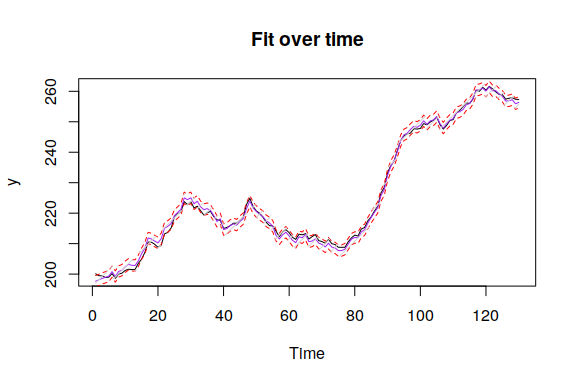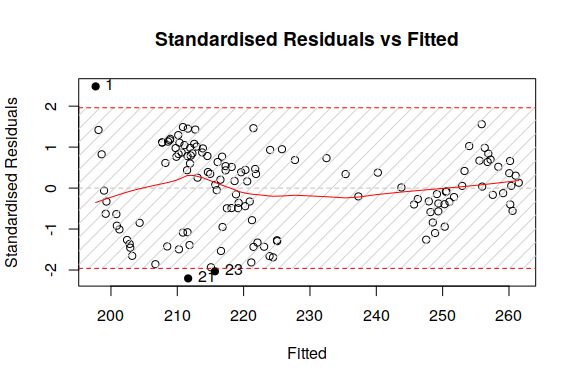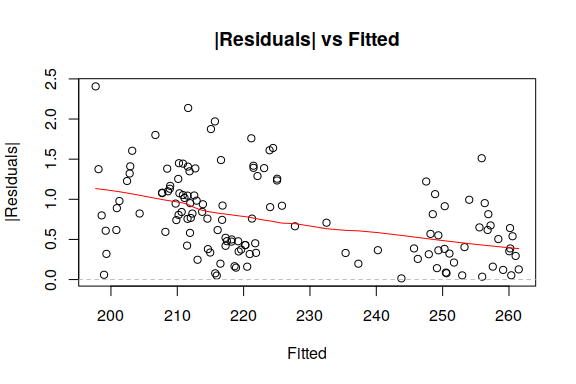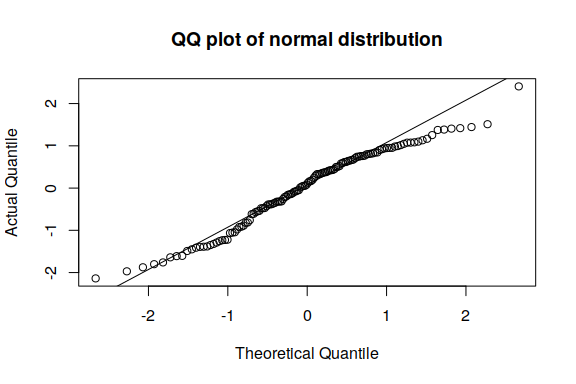Importance tells us how important the respective variable is in the combination. 1 means 100% important, 0 means not important at all.

And the forecast using the holdout sample:

ourForecast <- predict(ourModel,BJHoldout)
plot(ourForecast)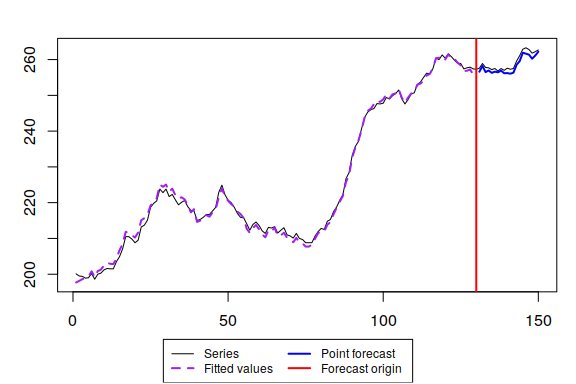These are the main functions implemented in the package for now. If you want to read more about IC model selection and combinations, I would recommend (Burnham and Anderson 2004) textbook.

## lmDynamic

This function is based on the principles of lmCombine() and point ICs. It allows not only combining the models but also to capture the dynamics of it parameters. So in a way this corresponds to a time varying parameters model, but based on information criteria.

Continuing the example from lmCombine(), let’s construct the dynamic model:

ourModel <- lmDynamic(BJInsample,bruteforce=FALSE)

We can plot the model and ask for the summary in the similar way as with lmCombine():

ourSummary <- summary(ourModel)
ourSummary
#> The pAICc combined model
#> Response variable: y
#> Distribution used in the estimation: Normal
#> Coefficients:
#>             Estimate Std. Error Importance Lower 2.5% Upper 97.5%
#> (Intercept)  20.9064     0.2029     1.0000    20.5048     21.3080
#> xLag5         2.3138     0.0982     0.9168     2.1194      2.5082
#> xLag10        1.4976     0.1097     0.8136     1.2804      1.7149
#> xLead5        0.0452     0.0225     0.2719     0.0007      0.0897
#> xLag3         4.8490     0.0715     0.9940     4.7075      4.9905
#> xLag4         3.4601     0.0786     0.9792     3.3045      3.6156
#> xLag8         1.3389     0.1052     0.7875     1.1306      1.5472
#> xLag7         1.2486     0.1089     0.7689     1.0331      1.4642
#> xLag6         1.6016     0.1082     0.8322     1.3874      1.8158
#> xLag9         1.2867     0.1058     0.7835     1.0772      1.4962
#> xLead10       0.1828     0.0372     0.5460     0.1091      0.2565
#>
#> Error standard deviation: 0.9698
#> Sample size: 130
#> Number of estimated parameters: 9.6935
#> Number of degrees of freedom: 120.3065
#> Approximate combined information criteria:
#>      AIC     AICc      BIC     BICc
#> 397.7097 399.4474 425.5062 429.7353
plot(ourModel)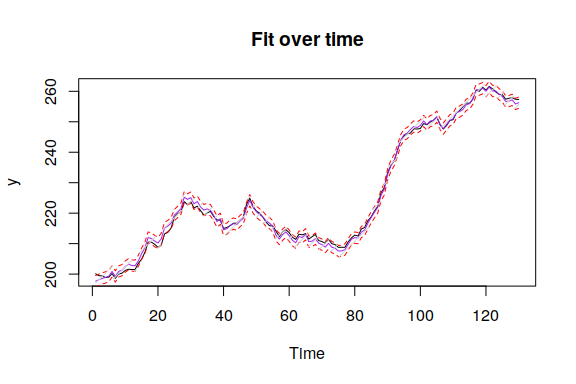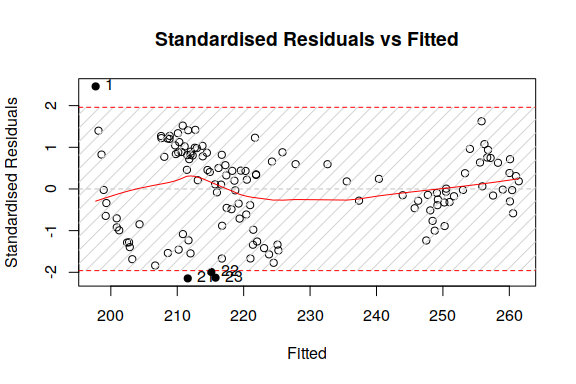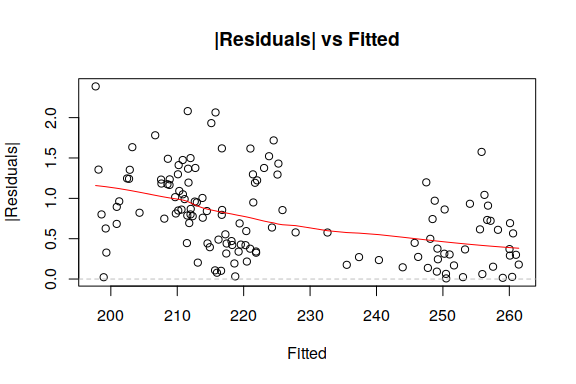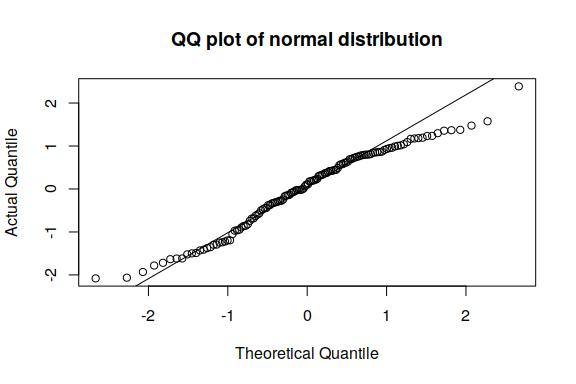The coefficients in the summary are the averaged out over the whole sample. The more interesting elements are the time varying parameters, their standard errors (and respective confidence intervals) and time varying importance of the parameters.

# Coefficients in dynamics
head(ourModel$coefficientsDynamic) #> (Intercept) xLag5 xLag10 xLead5 xLag3 xLag4 #> [1,] 23.60573 0.9124884 1.959955e-05 -0.721112230 5.951427 5.082177 #> [2,] 22.38598 2.8542174 2.639091e-04 -0.021414907 4.535744 3.565041 #> [3,] 20.77044 2.2786631 4.450298e-01 -0.050189258 4.604533 3.345463 #> [4,] 20.58782 2.3110223 1.536021e+00 0.011477495 4.723528 3.342884 #> [5,] 20.80873 2.3188685 1.563239e+00 0.277715293 4.696093 3.347107 #> [6,] 20.45115 2.3108629 1.548654e+00 -0.001522025 4.689835 3.334904 #> xLag8 xLag7 xLag6 xLag9 xLead10 #> [1,] 0.001624485 0.01123104 5.727901 8.938742e-05 0.58536793 #> [2,] 0.908322289 2.88163511 2.816300 1.258860e-02 0.12620560 #> [3,] 1.847760219 1.45439464 1.876770 1.660029e+00 0.36758831 #> [4,] 1.403875086 1.32014454 1.638111 1.293892e+00 0.27105325 #> [5,] 1.376152088 1.32850215 1.590832 1.315353e+00 0.02146607 #> [6,] 1.364634055 1.33933969 1.646731 1.295657e+00 0.33329320 # Standard errors of the coefficients in dynamics head(ourModel$se)
#> NULL
# Importance of parameters in dynamics
head(ourModel$importance) #> (Intercept) xLag5 xLag10 xLead5 xLag3 xLag4 #> [1,] 1 0.1507689 8.627419e-06 0.9679247 0.9858066 0.9493127 #> [2,] 1 0.9497745 1.038545e-04 0.2439662 0.9997646 0.9789154 #> [3,] 1 0.9994897 2.414643e-01 0.5148553 1.0000000 0.9999998 #> [4,] 1 1.0000000 9.999800e-01 0.1523935 1.0000000 1.0000000 #> [5,] 1 1.0000000 9.999930e-01 0.9013562 1.0000000 1.0000000 #> [6,] 1 1.0000000 9.994536e-01 0.1879295 1.0000000 1.0000000 #> xLag8 xLag7 xLag6 xLag9 xLead10 #> [1,] 0.0003704504 0.001949508 0.8925037 3.110388e-05 0.96266104 #> [2,] 0.2413684374 0.825770321 0.9145188 3.865992e-03 0.37708821 #> [3,] 0.9859379557 0.931731575 0.9937307 8.483688e-01 0.99955868 #> [4,] 0.9977832513 0.995198877 0.9998458 9.968067e-01 0.81082070 #> [5,] 0.9961713650 0.994667224 0.9990858 9.980981e-01 0.06424596 #> [6,] 0.9617665765 0.997275794 0.9996739 9.875884e-01 0.99940238 The importance can also be plotted using plot() and coef() functions, which might produce a lot of images: The plots show how the importance of each parameter changes over time. The values do not look as smooth as we would like them to, but nothing can be done with this at this point. If you want something smooth, then smooth these values out using, for example, cma() function from smooth package. In fact, even degrees of freedom are now also time varying: ourModel$dfDynamic
#>     6.911337  7.535136 10.515137 10.952829 10.953618 11.133090 10.474677
#>     9.840000  8.769821  8.601164  7.887939  8.494532  8.201896  8.893279
#>    7.300218  7.959795  8.627046  9.113996 10.080620  8.925524  7.867106
#>    9.020157 10.047444 10.493425  9.987738  9.806582 10.137245  9.146601
#>    9.045839 10.115637  8.601941  8.998909  8.657689  8.480871  9.907744
#>   10.425142 10.447090  9.728446 10.256308 10.995352 10.217130 11.127022
#>   10.648101 10.151455  9.978704  9.984659  8.993345  9.007418  9.986345
#>   10.635093 10.056903 11.097249  9.632913 10.052168 10.902700  9.099186
#>   10.004737  9.014318  9.036567  9.073067  8.773906  8.823669  8.952235
#>    9.180900 10.148184  8.904212  7.626750  8.987974  9.996634  8.893280
#>    8.915928  9.002316  9.215663  9.142042  9.350393  9.009053 10.322301
#>    9.453731 10.202903  9.530741 10.210279  9.061256 10.066453 10.286293
#>   11.127963 10.466767 10.273227  9.251079 10.004314  9.716571 10.134046
#>   10.526572 10.261545 11.101570  9.890747 10.628202  8.684585  9.925863
#>    9.705747  8.674943  9.245135 10.759633  9.954817 11.051003 10.482518
#>   9.999923 10.013871  9.627020 10.964018 10.083104 10.947717  9.942613
#>   9.387497  9.964373 11.010653 11.108702 11.015721 10.010086 10.597963
#>  10.160695 10.859131  9.975984  9.960376 11.019536 10.033024  9.240142
#>   9.047717  8.859129  9.038892  9.330330
ourModel\$df.residualDynamic
#>    123.0887 122.4649 119.4849 119.0472 119.0464 118.8669 119.5253 120.1600
#>    121.2302 121.3988 122.1121 121.5055 121.7981 121.1067 122.6998 122.0402
#>   121.3730 120.8860 119.9194 121.0745 122.1329 120.9798 119.9526 119.5066
#>   120.0123 120.1934 119.8628 120.8534 120.9542 119.8844 121.3981 121.0011
#>   121.3423 121.5191 120.0923 119.5749 119.5529 120.2716 119.7437 119.0046
#>   119.7829 118.8730 119.3519 119.8485 120.0213 120.0153 121.0067 120.9926
#>   120.0137 119.3649 119.9431 118.9028 120.3671 119.9478 119.0973 120.9008
#>   119.9953 120.9857 120.9634 120.9269 121.2261 121.1763 121.0478 120.8191
#>   119.8518 121.0958 122.3733 121.0120 120.0034 121.1067 121.0841 120.9977
#>   120.7843 120.8580 120.6496 120.9909 119.6777 120.5463 119.7971 120.4693
#>   119.7897 120.9387 119.9335 119.7137 118.8720 119.5332 119.7268 120.7489
#>   119.9957 120.2834 119.8660 119.4734 119.7385 118.8984 120.1093 119.3718
#>   121.3154 120.0741 120.2943 121.3251 120.7549 119.2404 120.0452 118.9490
#>  119.5175 120.0001 119.9861 120.3730 119.0360 119.9169 119.0523 120.0574
#>  120.6125 120.0356 118.9893 118.8913 118.9843 119.9899 119.4020 119.8393
#>  119.1409 120.0240 120.0396 118.9805 119.9670 120.7599 120.9523 121.1409
#>  120.9611 120.6697

And as usual we can produce forecast from this function, the mean parameters are used in this case:

ourForecast <- predict(ourModel,BJHoldout)
plot(ourForecast)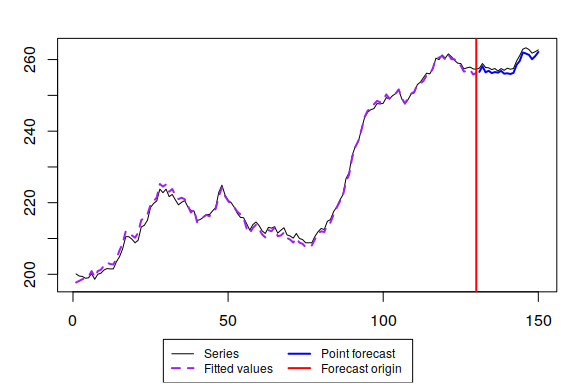This function is currently under development, so stay tuned.